# Embeddings of finite metric spaces in Hilbert space

In this post we discuss the following notion: Let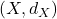andbe two metric spaces, one says thatembeds intowith distortionif there existsand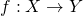such that for any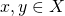,We write this as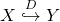.

Note that ifis a normed vector space (over the reals) then the scaling parameter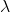in the above definition is unnecessary since one can always perform this scaling with the embedding(indeed in this case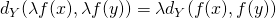).

Informally ifembeds intowith distortion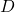then up to an ‘error’ of orderone can view(once mapped with the embedding) as a subset of. This is particularly useful whenhas a specific known structure that we may want to exploit, such as.

The caseis of particular interest since then one embeds into a Hilbert space which comes with a lot of ‘structure’. Thus we mainly focus on embeddings in, and we also focus onbeing a finite metric space with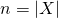.

First observe that the case(embeddings in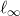) is trivial:

Proposition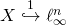Proof: Consider the embedding. It is obvious thatand the other side of the inequality follows from the triangle inequality for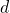. Thus one haswhich concludes the proof.Could it be that the situation is that simple for? It seems very unlikely becauseis a much more structured space than an arbitrary metric space. Consider for examplewith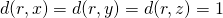and(that isis a tree with rootand three leaves). It is easy to see that it is impossible to embedintowith distortion. Indeed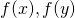andwould be on a Euclidean ball of radiusand they would be at distance 2 from each other, which is impossible for 3 points.

So what can we say in general about embeddings into? The following beautiful result gives the answer:

Theorem (Bourgain 1985)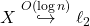It can be shown that this result is unimprovable in general. However for specific classes of finite metric spaces one can hope for some improvement and we refer to the survey by Linial for more details on this.

Embeddings inwith small distortion

While Bourgain’s theorem is a very strong statement in the sense that it applies to any finite metric space, it is also quite weak in the sense that the distortion is large, of order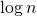. For some applications it is desirable to have a bounded distortion that does not grow with the size of, or even a distortion of order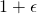with small. As we said previously the only way to obtain such a statement is to make further assumptions on. We will not take this route in this post but let me just mention the Johnson-Lindenstrauss lemma which should be well-known to Machine Learners and alike:

Lemma (Johnson-Lindenstrauss 1984) Letthen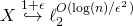.

Now what can we say in general about embeddings with small distortion into? There is a well-known result in convex geometry with the same flavor than this question, this is Dvoretzky’s theorem: any symmetric convex body in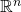has a section of dimensionwhich is Euclidean up to an error of order. The analogue for the theory of metric embeddings is called the non-linear Dvoretzky’s theorem and it goes as follows:

Theorem (Bourgain–Figiel–Milman 1986) Let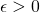. There existswith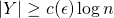and.

Again this theorem has a weakness: while the distortion is small it also only applies to a small subset of the original metric space (of size). In some sense we have now two extreme results, Bourgain’s theorem which applies to the entire metric space but with a large distortion, and Bourgain–Figiel–Milman which applies to a small small subset but with a small distortion. A natural question at this point is to try to ‘interpolate’ between these two results. Such a statement was first obtained by Bartal, Linial, Mendel and Naor (2002) and later improved in Mendel and Naor (2006):

Theorem (Mendel and Naor 2006) Let. There existswithand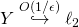.

In future posts I will try to prove some of these theorems. This might take a while as in the coming weeks I will be traveling to various conferences (including COLT 2013 and ICML 2013) and I will try to blog about some of the stuff that I learn there.

This entry was posted in Theoretical Computer Science. Bookmark the permalink.